# Small Transversals in Partitionable Graphs

Following Bland, Huang, and Trotter [ MR 80g:05034];[ MR 86e:05075] a graph is called partitionable if, for some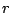and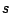, it has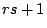vertices and, no matter which vertex is removed, the set of the remaining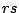vertices can be partitioned intopairwise disjoint cliques of sizeand also intopairwise disjoint stable sets of size. Odd holes and odd antiholes are partitionable; many additional partitionable graphs have been constructed by V. Chvátal, R. L. Graham, A. F. Perold, and S. H. Whitesides [ MR 81b:05044].

A small transversal in a graph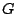is a set of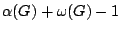vertices which meets all cliques of size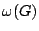and all stable sets of size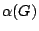. The following problem is an easier variation on a conjecture contributed to the 1993 [workshop on perfect graphs] by Gurvich and Temkin and on two conjectures proposed by Bacso, Boros, Gurvich, Maffray, and Preissmann [ MR 2000h:05116].

Conjecture. Every partitionable graphwith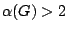and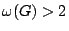has a small transversal or else contains a hole of length five.

One of the milestones in the development of our understanding of perfect graphs was the theorem of Lovasz [46 #8885], asserting that every minimal imperfect graphhas precisely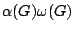+1 vertices. This theorem implies that every minimal imperfect graph is partitionable and that - as pointed out by Chvatal [ MR 86h:05091] - no minimal imperfect graph contains a small transversal. It follows that a proof of the conjecture would provide another proof of the Strong Perfect Graph Theorem.

A partitionable graph without a small transversal has been constructed by by Chvatal, Graham, Perold, and Whitesides (op.cit.). Its vertices are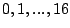; vertices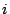and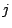are adjacent if and only if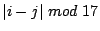is one of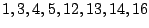.

Ara Markosian claims [here] that this is the only known partitionable graph without a small transversal. One of the many holes of length five in this graph is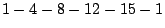Additional information on related results and problems can be found [here]

Contributed by Vasek Chvatal

Back to the main index for Perfect Graphs.